# NCERT Solutions class 12 physics Dual Nature of Radiation and Matter Part 1## myCBSEguide App

CBSE, NCERT, JEE Main, NEET-UG, NDA, Exam Papers, Question Bank, NCERT Solutions, Exemplars, Revision Notes, Free Videos, MCQ Tests & more.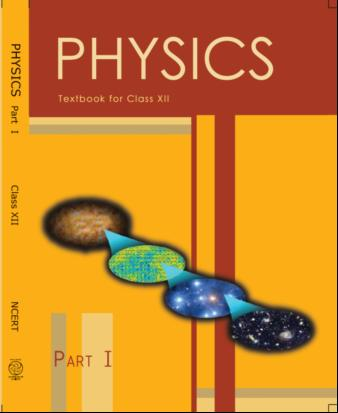## NCERT Class 12 Physics Chapter-wise Solutions

• 1 – Electric Charges and Fields
• 2 – Electrostatic Potential and Capacitance
• 3 – Current Electricity
• 4 – Moving Charges and Magnetism
• 5 – Magnetism and Matter
• 6 – Electromagnetic Induction
• 7 – Alternating Current
• 8 – Electromagnetic Waves
• 9 – Ray Optics and Optical Instruments
• 10 – Wave Optics
• 11 – Dual Nature of Radiation and Matter
• 12 – Atoms
• 13 – Nuclei
• 14 – Semiconductor Electronic: Material, Devices and Simple Circuits
• 15 – Communication Systems

## CHAPTER 11 DUAL NATURE OF RADIATION AND MATTER

• 11.1 Introduction
• 11.2 Electron Emission
• 11.3 Photoelectric Effect
• 11.4 Experimental Study of Photoelectric Effect
• 11.5 Photoelectric Effect and Wave Theory of Light
• 11.6 Einstein’s Photoelectric Equation: Energy Quantum of Radiation
• 11.7 Particle Nature of Light: The Photon
• 11.8 Wave Nature of Matter
• 11.9 Davisson and Germer Experiment

## NCERT Solutions class 12 physics Dual Nature of Radiation and Matter Part 1

1: Find the

(a) maximum frequency, and

(b) minimum wavelength of X-rays produced by 30 kV electrons.

2: The work function of caesium metal is 2.14 eV. When light of frequency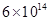Hz is incident on the metal surface, photoemission of electrons occurs. What is the

(a) maximum kinetic energy of the emitted electrons,

(b) Stopping potential, and

(c) maximum speed of the emitted photoelectrons?

3: The photoelectric cut-off voltage in a certain experiment is 1.5 V. What is the maximum kinetic energy of photoelectrons emitted?

4: Monochromatic light of wavelength 632.8 nm is produced by a helium-neon laser. The power emitted is 9.42 mW.

(a) Find the energy and momentum of each photon in the light beam,

(b) How many photons per second, on the average, arrive at a target irradiated by this beam? (Assume the beam to have uniform cross-section which is less than the target area), and

(c) How fast does a hydrogen atom have to travel in order to have the same momentum as that of the photon?

5: The energy flux of sunlight reaching the surface of the earth is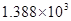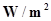. How many photons (nearly) per square metre are incident on the Earth per second? Assume that the photons in the sunlight have an average wavelength of 550 nm.

6: In an experiment on photoelectric effect, the slope of the cut-off voltage versus frequency of incident light is found to be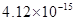V s. Calculate the value of Planck’s constant.

7: A 100 W sodium lamp radiates energy uniformly in all directions. The lamp is located at the centre of a large sphere that absorbs all the sodium light which is incident on it. The wavelength of the sodium light is 589 nm. (a) What is the energy per photon associated with the sodium light? (b) At what rate are the photons delivered to the sphere?

8: The threshold frequency for a certain metal is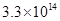Hz. If light of frequency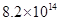Hz is incident on the metal, predict the cutoff voltage for the photoelectric emission.

9: The work function for a certain metal is 4.2 eV. Will this metal give photoelectric emission for incident radiation of wavelength 330 nm?

10: Light of frequency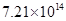Hz is incident on a metal surface. Electrons with a maximum speed of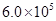m/s are ejected from the surface. What is the threshold frequency for photoemission of electrons?

11:  Light of wavelength 488 nm is produced by an argon laser which is used in the photoelectric effect. When light from this spectral line is incident on the emitter, the stopping (cut-off) potential of photoelectrons is 0.38 V. Find the work function of the material from which the emitter is made.

12: Calculate the

(a) momentum, and

(b) de Broglie wavelength of the electrons accelerated through a potential difference of 56 V.

13: What is the

(a) momentum,

(b) speed, and

(c) de Broglie wavelength of an electron with kinetic energy of 120 eV.

14: The wavelength of light from the spectral emission line of sodium is 589 nm. Find the kinetic energy at which

(a) an electron, and

(b) a neutron, would have the same de Broglie wavelength.

15:  What is the de Broglie wavelength of

(a) a bullet of mass 0.040 kg travelling at the speed of 1.0 km/s,

(b) a ball of mass 0.060 kg moving at a speed of 1.0 m/s, and

(c) a dust particle of mass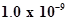kg drifting with a speed of 2.2 m/s?

16: An electron and a photon each have a wavelength of 1.00 nm. Find

(a) their momenta,

(b) the energy of the photon, and

(c) the kinetic energy of electron.

17: (a) For what kinetic energy of a neutron will the associated de Broglie wavelength be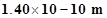?

(b) Also find the de Broglie wavelength of a neutron, in thermal equilibrium with matter, having an average kinetic energy of (3/2) kT at 300 K.

18:  Show that the wavelength of electromagnetic radiation is equal to the de Broglie wavelength of its quantum (photon).

## NCERT Solutions for Class 12 Physics

NCERT Solutions Class 12 Physics PDF (Download) Free from myCBSEguide app and myCBSEguide website. Ncert solution class 12 physics includes text book solutions from both part 1 and part 2. NCERT Solutions for CBSE Class 12 Physics have total 20 chapters. 12 Physics NCERT Solutions in PDF for free Download on our website. Ncert physics class 12 solutions PDF and physics ncert class 12 PDF solutions with latest modifications and as per the latest CBSE syllabus are only available in myCBSEguide

To download NCERT Solutions for class 12 Physics, Chemistry, Biology, History, Political Science, Economics, Geography, Computer Science, Home Science, Accountancy, Business Studies and Home Science; do check myCBSEguide app or website. myCBSEguide provides sample papers with solution, test papers for chapter-wise practice, NCERT solutions, NCERT Exemplar solutions, quick revision notes for ready reference, CBSE guess papers and CBSE important question papers. Sample Paper all are made available through the best app for CBSE students and myCBSEguide website.